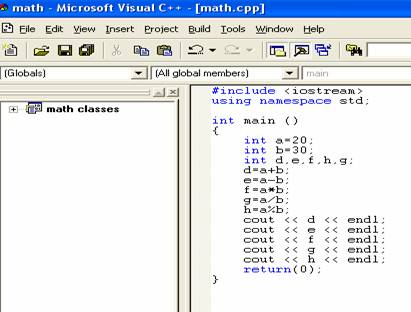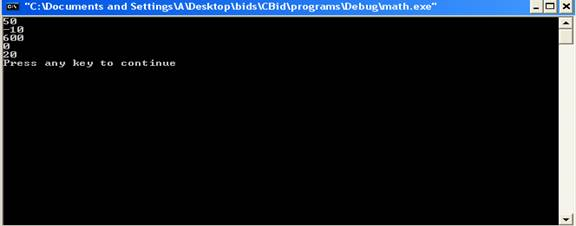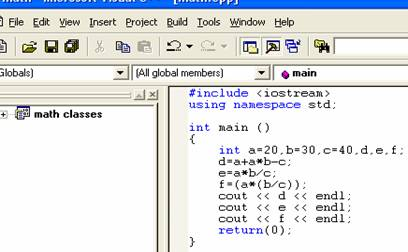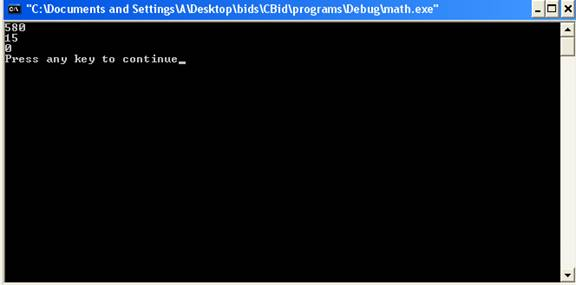Math Operations

Before reading this tutorial, you should learn about variables and input values using cin.

In any language, there are some operators to perform arithmetic, logical and control operations.

The basic operators which are used to perform arithmetic operations on integers are as follows:-

Operator                                              Operation

• Subtraction, also called unary subtraction

*                                                        Multiplication

/                                                         Division

%                                                       Modulus (remainder after division)

++                                                      Increment

--                                                       Decrement

The operators +,-,* and / perform the same operation as they do in other languages.  The operators + and – are unary operators and can take one or two operands. The multiply and divide are called binary operators as they take two operands.

Integer division will always give the result which is of integer type and truncates the remainder. The output of integer division will be the quotient. For example, 5/2 will give the answer 2 and not 2.5. The modulus operator gives the remainder after the integer division. For example, 5/2 will give the answer 1, which is the remainder. Here is a program which illustrates the functioning of the operators.The result is: -When the statement

d=a+b; is executed, it takes the values 20 and 30 of variables a and b respectively, performs their sum and assigns the result to variable d which gets the value 50.

Similarly, statement

e=a-b;  gives the result -10,

f=a*b;  gives the result 600, where integer variable a is equal to 20 and variable b is equal to 30.

The output of statement

h=a% b operator is 20 as it is modulo operator which gives the remainder when a is divided by b.

The output of division operator is 0 as the quotient of 20/30 is 0.

Note here that all the answers are in integers as we have defined the variables to be of type int. If we define them to be of type float or double, then the answers would be different. Also, modulo operator (%) does not works with float and double.

PRECENDENCE

In an expression which involves several operators, the order in which the operators are evaluated depends on the priority given to them. The priority given to the operators is called precedence. The operators such as *, /, % have higher precedence than the operators +,-. The operators *, / and % have same precedence and operators + and - have same precedence. For example, in the statement

a+b*c;  b*c is evaluated before and the result of b*c is added to a. It is same as

(a+(b*c));

ASSOCIATIVITY

If the expression contains operators of same precedence then the order in which they are evaluated can be either left associative or right associative. Left associative means that left most operators are evaluated first then the operators on the right. Right associative means that right most operators are evaluated first than the operators on the left. If the expression a+b+c is evaluated in the left associative manner, then result of a+b is added to c. Similarly in the right associative, result of b+c is added to a.

PARENTHESES

If the expression contains parentheses, then the natural order of execution of the operators is overridden by the parentheses. The parentheses are given more priority than the any of the operators. Parentheses force the operations to have higher precedence. If the expression contains nested parentheses, then the innermost parentheses are evaluated first. For example in the expression a*(b+c), b+c is evaluated before the multiplication operation.

Here is the program which illustrates the meaning of precedence, associativity and parentheses.The result of the program isIn the given program a=20, b=30 and c=40. In the statement

a+a*b-c;

a*b is evaluated first then the operators + and – are evaluated. The operation a*b gives 600 and then a is added and c is subtracted which gives the final result 580.

In the statement a*b/c, the operators * and / have same precedence and it is left associative. The multiplication operator is evaluated before the division operator. The operation a*b gives the result 600 which is then divided by 40 and giving the final result as 15.

In the last statement parentheses are used, the innermost expression (b/c) is evaluated first giving the result 0 where b=20 and c=40, which is then multiplied with variable a and the final result is 0.

INCREMENT, DECREMENT OPERATOR

The operator ++ and -- are called an increment operator and decrement operator respectively. They are unary operators and are applied to integers. They perform the same function as x=x+1 in the case of increment operator and performs the function x=x-1 in the case of decrement operator. Increment operator increments the value of variable x by 1 whereas decrement operator decreases the value of variable x by 1.  For example ++x and x=x+1 have same effect. These operators are more precise and directly modify the values. If the operator is written in front of the variable then it is called prefix form whereas if the operator is written after the variable then it is called postfix form. Prefix form and postfix form have different meanings.

Prefix form - ++x. It means that value of variable x is incremented before the value is used in context. For example, z=++x; when x=6 gives z=7 and x=7. Same is the case with decrement operator for example, the expression z=--x when x=6 gives z=5 and x=5.

Postfix form - x++. It means that value of variable x is incremented after the value is used in context. For example z=x++, when x=6 gives z=6 and x=7.  Same is the case with decrement operator for example, the expression z=x--    when x=6 gives z=6 and x-5.

The op= ASSIGNMENT OPERATORS

These operators consist of an operator sign and equality operator. They modify the current value of the variable by performing an operation. Consider the following case

x +=2; is same as x=x+2;

x *=2 ; performs the operation x = x * 2 and gives the value 6 when the original value of x was 3.

Basically these operators are short hand notations for the normal operators where the meaning is that the operation specified in the statement has to be performed on the variable left to the operator with the variable right to the equality operator and the value is stored in the variable left to the operator.

Let us discuss the next tutorial on control structures in C++.

Go to the previous lesson or proceed to the next lesson